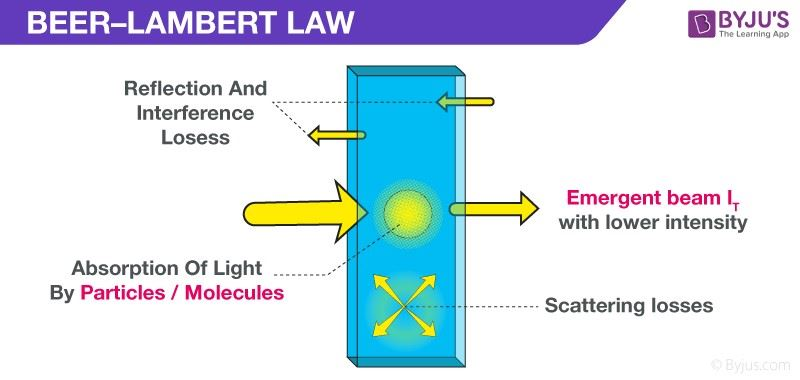# Absorbance vs Concentration

## Introduction

According to Beer’s Law, A = εLc, a substance’s concentration and absorbance are directly proportional under ideal conditions: a high-concentration solution absorbs more light. In comparison, a low-concentration solution absorbs less light. Beer’s Law allows you to determine an unknown phosphate concentration after determining the absorbance because concentration and absorbance are proportionate.

The concentration (c) of a sample is one factor that affects its absorbance. As the concentration rises, more radiation should be absorbed, increasing the absorbance. As a result, the concentration and absorbance are directly proportional.

## Beer’s Law also called Lambert-Beer Law or Beer-Lambert Law

Beer’s Law is an equation that relates light attenuation to material qualities. According to the law, the concentration of a chemical is directly proportional to the absorbance of a solution. Using a colorimeter or spectrophotometer, the relationship can determine the concentration of a chemical species in a solution.

The Beer-Lambert Law, the Lambert-Beer Law, and the Beer-Lambert–Bouguer Law are all variations of Beer’s Law. There are so many names because there are multiple laws involved.### The Equation for Beer’s Law

Beer’s Law can be summed up this way:

A = εLc

where,

• A is the amount of light that the sample absorbs at a specific wavelength.
• ε is the extinction coefficient at the molar level
• L is the length of time that light travels through the solution.
• The concentration of the absorbing species is denoted by c.

### Beer’s Law: How to Use It

While many current devices calculate Beer’s Law by comparing a blank cuvette to a sample, it’s simple to create a graph using standard solutions to establish a specimen’s concentration. For dilute solutions, the graphing approach assumes a straight-line relationship between absorbance and concentration.

### Beer’s Law’s Importance

In the fields of chemistry, physics, and meteorology, Beer’s Law is very essential. In chemistry, Beer’s Law is used to determine the concentration of chemical solutions, assess oxidation, and monitor polymer deterioration. The attenuation of radiation through the Earth’s atmosphere is similarly described by the law. While the rule is most commonly associated with light, it also aids scientists in understanding the attenuation of particle beams such as neutrons.

## Factors that Influence the Absorbance

The concentration (c) of a sample is one factor that affects its absorbance. As the concentration rises, more radiation should be absorbed, increasing the absorbance. As a result, the concentration and absorbance are directly proportional.

The length of the path (b) is a second consideration. The longer the path length, the more molecules in the path of the radiation beam, and thus the absorbance increases. As a result, the length of the path is proportional to the concentration.

The molar absorptivity (ε) is the third factor when the concentration is expressed in moles/litre and the route length is expressed in centimetres. This is more commonly referred to as the extinction coefficient in some sectors of study.

We may construct the Beer-Lambert law (commonly referred to as Beer’s Law) to show this relationship because concentration, path length, and molar absorptivity are all directly proportional to absorbance.

## Calculation of Concentration from Absorbance

The maximum absorbance value of a sample is known to be 275 nm. 8400 M-1cm-1 is its molar absorptivity. The cuvette is 1 cm in diameter. A = 0.70 is found using a spectrophotometer. What is the sample’s concentration (c)?

To solve the problem, we use Beer’s Law:

i.e., A = εLc

⇒ 0.70 = (8400 M-1cm-1)(1 cm)(c)

Dividing both sides of the equation by [(8400 M-1 cm-1)(1 cm)],

c = 8.33 x 10-5 mol/L

## Frequently Asked Questions on Absorbance vs Concentration

Q1

### What is the significance of Beer’s law in chemistry?

In the domains of chemistry, physics, and meteorology, Beer’s Law is very essential. In chemistry, Beer’s Law is used to determine the concentration of chemical solutions, assess oxidation, and monitor polymer deterioration. The attenuation of radiation through the Earth’s atmosphere is similarly described by the law.

Q2

### What’s the difference between calibration curve and concentration curve?

The main distinction between a calibration curve and a concentration curve is that a calibration curve is a graph of absorbance and concentration, whereas concentration is the amount of a chemical distributed in a unit volume.

Q3

### What effect does concentration have on absorbance?

Absorbance is proportional to the substance’s concentration. The absorbance of a substance is proportional to its concentration. Because the number of molecules with which light interacts influences the quantity of light that is absorbed, this is the case.

Q4

### Is wavelength a factor in absorbance?

Beer’s Law states that the absorbance is proportional to the concentration of absorbing material at constant path length, where L is the path length, c is the concentration, and A is a constant that varies depending on the wavelength of the light, the absorbing material, and the medium (solvent and other components).

Q5

### What is the practical use of Beer’s law?

The type of poison can be identified by comparing the spectra of probable toxins to those found at the crime scene. Once the poison’s identity has been verified, Beer’s law can be used to calculate the poison concentration in a poisoned wine.

Take up a quiz on Absorbance vs Concentration!